# Creating Laws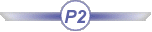This task shows how to create evolution laws within a .CATPart document, to be used later on when creating Generative Shape Design elements, such as swept surfaces, or parallel curves.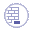Open the Law1.CATPart document.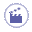1. Click Law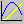.

 The Law Definition dialog box appears.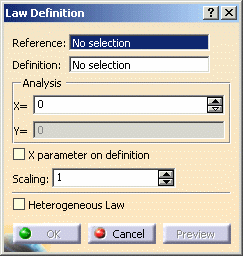2. Select the reference line.

3. Select a definition curve.

The law is computed as the distance between points on the reference line and their matching points onto the curve.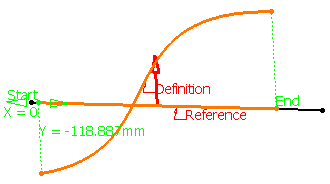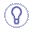• Laws can be created using negative values. The intersection between the reference line and the definition curve is taken into account to change the law evaluation sign. The direction lets allows you to choose which side of the reference line must be considered as positive.
• If X parameter on definition is checked, the X parameter is displayed on the selected curve and represents the percentage of the curvilinear abscissa on this curve. The law is computed by projecting the start point normally onto the reference line.
• You can analyze the law using the manipulator, or specifying a value in the X field. This parameter represents the percentage of the curvilinear abscissa on this curve. The law is computed by projecting the start point normally onto the reference line.
The Y field indicates the distance between any point on the reference line and its matching point on the selected curve.

4. Define the law amplitude by entering a value or using the graphic manipulators in the Scaling field.

 When the law is applied to a geometric element, the latter usually is not of the same length as the reference line. Therefore a linear mapping is applied between the reference line and the element the law is applied to, resulting in a scaling of the law. In the illustration, the law is applied to a circular sweep (top) and to a parallel curve (bottom). The dotted lines represent the linear mapping between the law (middle) and the two elements to which it is applied.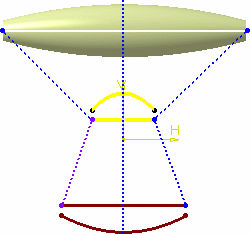5. Check Heterogeneous Law to define the applied law unit (none for ratio law; degree, radian, or grade for angle law) and the distance measure units (current unit by default).

 Two conversions will be performed during the law evaluation: conversion from the model unit (millimeters) to the stored measure unit conversion from the stored applied law unit to the model unit (degrees) in case of an angle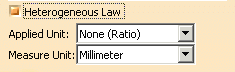6. Click OK to create the law.

 The law (identified as Law.xxx) is added to the specification tree. It is now ready for use in the creation of other Shape Design elements.
7. Click Parallel Curve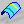.

8. In the Parallel Curve Definition dialog box, click Law....

9. Select the Law.1 from the specification tree.

10. Click OK.

The law is applied to the selected element.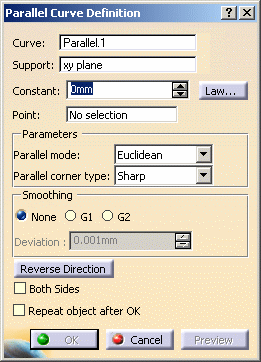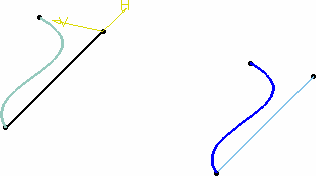• When the reference line and definition curve do not present the same length, only the common area is used to compute the law.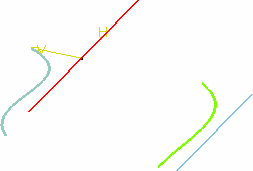• Check Both Sides to generate a parallel curve symmetrically on each side of the selected curve. Note that depending on the geometry, the elements may not appear symmetrical. They are if the curve is a line, otherwise, the resulting curves' shape may differ: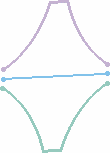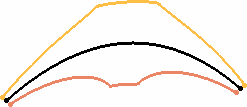Resulting parallel curves when a line is selected Resulting parallel curves when any curve is selected
• When X parameter on definition is deselected, the selected curve should not present several intersections with the plane normal to the reference line. If there are several intersections, the law cannot be evaluated and cannot be applied when creating geometric elements.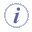Laws created using the Knowledge Advisor product, being mathematical formulas, can be used with Generative Shape Design's operators, such as swept surfaces, or parallel curves for instance.
For further information, refer to Creating and Using a Knowledge Advisor Law in Knowledge Advisor's User's Guide.
Note that laws created using the Law icon of Generative Shape Design product can be referenced by laws created with Knowledge Advisor product.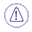Selecting a published law from another part is not allowed.